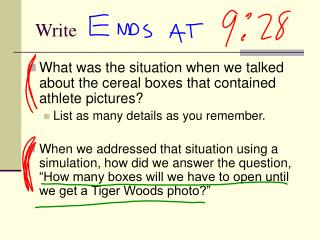# Write - PowerPoint PPT PresentationDownload PresentationWrite

Write
Download Presentation## Write

- - - - - - - - - - - - - - - - - - - - - - - - - - - E N D - - - - - - - - - - - - - - - - - - - - - - - - - - -
##### Presentation Transcript

1. Write • What was the situation when we talked about the cereal boxes that contained athlete pictures? • List as many details as you remember. • When we addressed that situation using a simulation, how did we answer the question, “How many boxes will we have to open until we get a Tiger Woods photo?”

2. Quiz Return • Mostly really good!

3. Finding Tiger

4. Well, simulations are great, but… • The law of large numbers tells us that, as we simulate more, the value of the response variable will approach the true mean. • Soooooo, what if we want to know that true mean?

5. A word on Bernoulli… • Jakob Bernoulli (Basel, December 27, 1654 - August 16, 1705), also known as Jacob, Jacques or James Bernoulli was a Swiss mathematician and scientist and the older brother of Johann Bernoulli. • He did not codify Bernoulli’s Principle, which is important – his nephew Daniel did. • He did work with Lebniz to shape up some of his early calculus. • He’s one of my favorite mathematicians, but he’s not pretty.

6. Bernoulli Trials! • A Bernoulli trial is just a particular type of situation, one which happens a lot: • there are exactly two possible outcomes • success • failure • the probability of success (called p) is constant • the trials are independent • When we’re dealing with a situation like this, computing probabilities is pretty easy.

7. Terms p = probability of success q = probability of failure • of course, q = 1-p

8. The Geometric Model • Consider the question, “In a Bernoulli trial situation, what is the probability that we will have our first success on the Nth trial?” • We answer this question using what is called the geometric model.

9. The Geometric Model • The probability that the first success will occur on trial X is equal to P(X) = qx-1p • μ = 1/p • σ = (q/p2)

10. So let’s simulate first. • Tiger is in 20 % of boxes. • Let’s get random numbers, and use 1-20 to mean Tiger. • Our response variable is the number of trials it takes to get a Tiger picture. • We’ll each run 5 trials.

11. Now, let’s compute the number of boxes this should take. • p = 0.2 • Find q. • Find the expected value of X – that is, the number of boxes it should take to find Tiger, using • μ = 1/p • Then, find the standard deeeeev. • σ = (q/p2)

12. Practice Again. • A basketball player makes 80 % of his foul shots. Assuming independence (as usual), find the probability that in tonight’s game… • misses for the first time on his fifth attempt. • makes his first basket on the fourth shot. • makes his first basket on his first, second, or third shots. • What is the expected number of shots it should take before he misses?

13. Practice Again Again. • Only 4 % of people have type AB blood. • How many people should we expect to have to check before we find one? • What’s the probability that the first AB we find will be the 8th person? • What’s the probability that we don’t find an AB until the 40th person?

14. Summary • Bernoulli trials have three qualities: • There are two possible outcomes. • The probability of success doesn’t change. • The trials are independent. • A geometric model uses Bernoulli trials to estimate the number of trials before success. • μ = 1/p • σ = (q/p2)

15. Homework Page 337 # 2 and 8